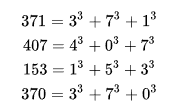# How to determine whether a number is an Armstrong number in C

In the number theory, an Armstrong number or named as well a narcissistic number, a pluperfect digital invariant and plus perfect number is basically a number that is the sum of its own digits each raised to the power of the number of digits. This definition depends on the base b of the number system used, e.g., b = 10 for the decimal system or b = 2 for the binary system (in base 2, the only narcissistic numbers are 0 and 1.):In this article, we'll show you a briefly explanation of how to determine if a number is an armstrong number in C.

## Implementation in C

In the following snippet, we will be reading the integer number provided by the user to verify if it's an armstrong number or not. We will use a while loop to check if the value of the number variable is not 0. If this condition is true, we'll use the first loop to determine at what power the number would be raised dividing a variable that contains the copy of the number provided by the user until it's 0 (as the `copyNumber` is declared as integer, the value will be automatically parsed as int e.g 0.15 becomes 0, exiting the loop).

The `remaining` variable will store the modulus of the value of number by 10 and `raised` will store the value of the remaining raised to the `powerNumber` using `pow()`. Finally, the last "if" condition statement is used to check both the value of `numberCopy` and `raised` are equal. If the condition is true, then it will print Armstrong number. Otherwise, it will execute the else condition statement and print not Armstrong number:

``````#include<stdio.h>
#include<math.h>

int main()
{
int number, raised = 0, powerNumber = 0, remaining, numberCopy;
printf("Enter any number: ");

scanf("%d",&number);

numberCopy = number;

while(numberCopy != 0){
numberCopy = numberCopy/10;
powerNumber++;
}

numberCopy = number;

while(number!=0)
{
remaining = number % 10;
raised += pow(remaining, powerNumber);
number = number/10;
}

if(numberCopy == raised){
printf("The given number is an armstrong number");
}else{
printf("The given number is not an armstrong number");
}

return 0;
}``````

If you compile the code, the console will start and will prompt for a number to the user. You can run the following examples:

• 1135: The given number is not an armstrong number
• 407: The given number is an armstrong number

Happy coding !